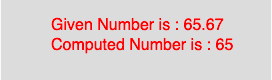# p5.js | floor() function

• Last Updated : 09 Apr, 2019

The floor() function in p5.js is used to calculate the floor value of a number. This function maps to the Math.floor() of javascript. It calculates the closest int value that is less than or equal to the value of the parameter.

Syntax

Hey geek! The constant emerging technologies in the world of web development always keeps the excitement for this subject through the roof. But before you tackle the big projects, we suggest you start by learning the basics. Kickstart your web development journey by learning JS concepts with our JavaScript Course. Now at it's lowest price ever!

```floor(number)
```

Parameters: The function accepts only one parameter as mentioned above and described below:

• number : This parameter stores the number to compute.

Below program illustrates the floor() function in p5.js:
Example:

 `function` `setup() {`` ` `    ``//create Canvas of size 270*80  ``    ``createCanvas(270, 80);``}`` ` `function` `draw() {``    ``background(220);``    ``//initialize the parameter  ``    ``let x = 65.67;``    ``//call to floor() function  ``    ``let y = floor(x);``    ``textSize(16);``    ``fill(color(``'red'``));``    ``text(``"Given Number is : "` `+ x, 50, 30);``    ``text(``"Computed Number is : "` `+ y, 50, 50);``}`

Output:Reference: https://p5js.org/reference/#/p5/floor

My Personal Notes arrow_drop_up